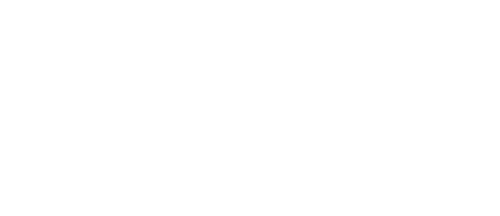MCC will be closed Monday, February 17th in observance of Presidents' Day. Offices and classes will resume on Tuesday, February 18th.
Apply Now!# MATH090M - Foundations for College Mathematics (3-0-3)

This course is designed to review and enhance mastery of basic mathematical and algebraic skills needed to complete a college level course in mathematics. Topics covered are operations with whole numbers, fractions, mixed numbers, decimals, signed numbers, percent, ratios, proportions, algebraic expressions, linear equations/ inequalities, exponents, square roots, and polynomials. Students will work with basic geometric formulas and basic descriptive statistics. Students will also manipulate formulas; convert between different units of measure; solve word problems; interpret/ analyze data; perform basic graphing techniques; perform operations with real numbers and polynomials; graph linear equations; solve linear equations, inequalities, linear- equation systems and quadratic equations; factor polynomials; and recognize basic functions and their related notations. Calculators will not be used in this course until the end of the term. This course is enhanced with web-based technology enabling self- paced learning. Students may take this course in multiple semesters until all concepts have been mastered. Course offered every semester. Credits do not count toward degree requirements. Successful completion of this course requires a grade of “C” or better.

Prerequisites: Placement Test or permission from the Mathematics Program Coordinator, the Department Chair, or a full time mathematics faculty member.